# Self-Training综述

## Introduction

Self-training 是最简单的半监督方法之一，其主要思想是找到一种方法，用未标记的数据集来扩充已标记的数据集。算法流程如下：

1. 首先，利用已标记的数据来训练一个好的模型，然后使用这个模型对未标记的数据进行标记。

2. 然后，进行伪标签的生成，因为已训练好的模型对未标记数据的所有预测都不可能都是完全正确的，因此对于经典的 Self-training，通常是使用分数阈值（confidence score）过滤部分预测，以选择出未标记数据的预测标签的一个子集。

3. 其次，将生成的伪标签与原始的标记数据相结合，并在合并后数据上进行联合训练。

4. 整个过程可以重复 n 次，直到达到收敛。

Self-training 最大的问题在就在于伪标签非常的 noisy，会使得模型朝着错误的方向发展。以下文章大多数都是为了解决这个问题。

## Paper

### Confidence Regularized Self-Training

1. ICCV 2019, https://kami.app/XxVJqozf8md0, https://github.com/yzou2/CRST
2. 这篇文章通过对模型进行正则化，迫使输出的 vector 不那么 sharp（参考 label smooth 的作用），从而减轻使用软伪标签学习的伪标签不正确或模糊所带来的误导效果。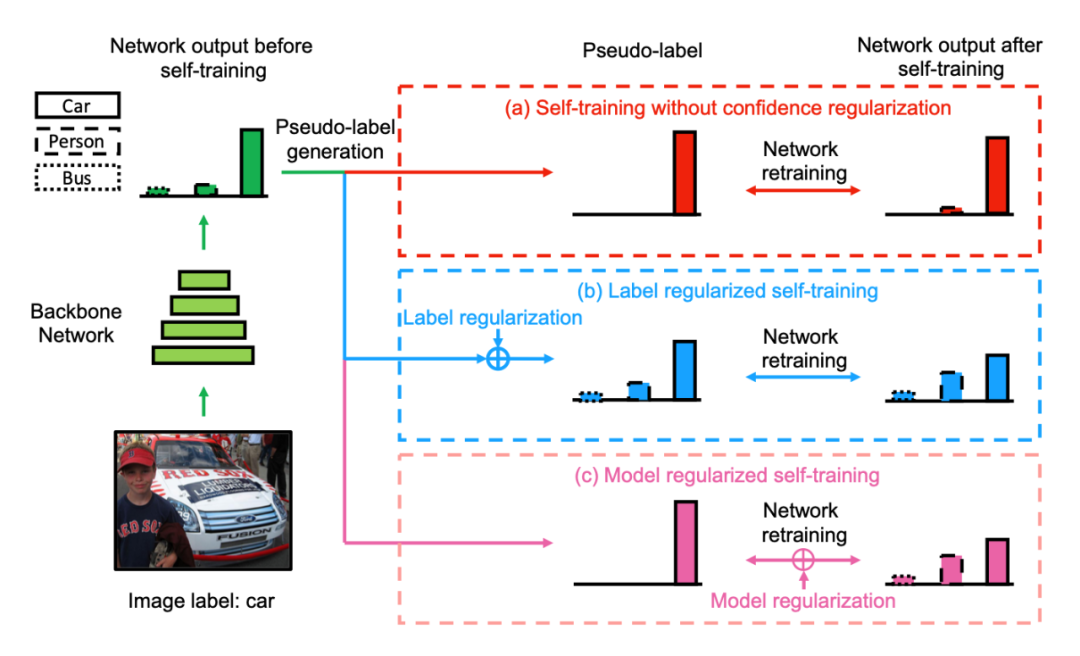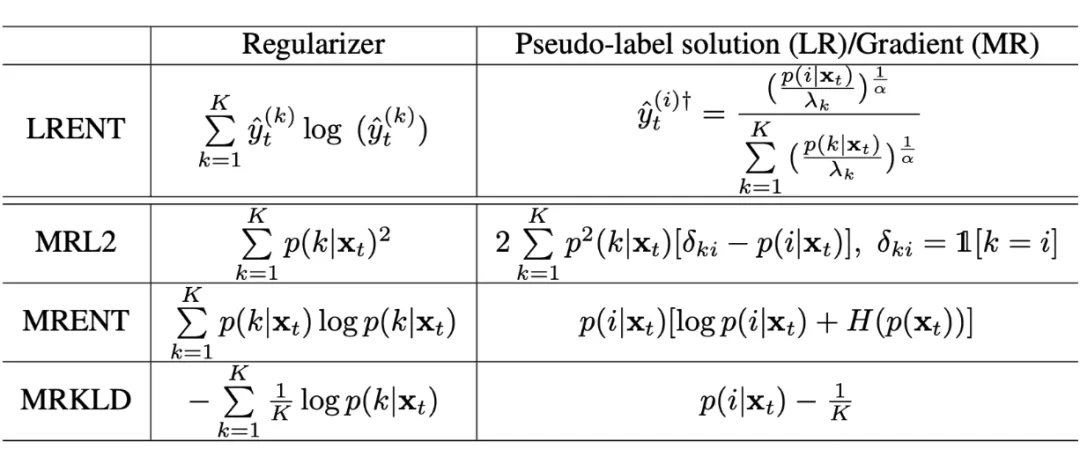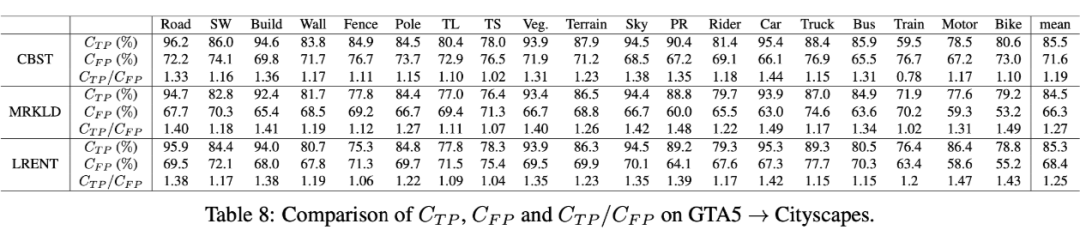### Uncertainty-aware Self-training for Text Classification with Few Labels

1. MSR，NIPS2020，https://github.com/microsoft/UST
2. 核心贡献：使用贝叶斯不一致主动学习（Bayesian Active Learning by Disagreement, BALD）的思想评估样本标签的不确定性，利用不确定性选择用于 re-train 模型的伪标签样本。

#### Background: Bayesian neural network(BNN)

$$p(y=c \mid x)=\int_{W} p\left(y=c \mid f^{W}(x)\right) p(W \mid X, Y) d W$$
$f$ 即 NN，这个积分显然是 intractable，因此就有很多工作用到了随机正则化的工具（dropout）来构建易于处理的分布族中 $q_{\theta}(w)$，它可以替代难以计算的真实模型后验。如果我们使用不同的 dropout 采样T个 masked model $\left\{\widetilde{W}_{t}\right\}_{t=1}^{T} \sim q_{\theta}(W)$，那么近似后验可以通过 MC 积分得到：
\begin{aligned} p(y=c \mid x) & \approx p\left(y=c \mid f^{W}(x)\right) q_{\theta}(W) d W \\ & \approx \frac{1}{T} \sum_{t=1}^{T} p\left(y=c \mid f^{\widetilde{W}_{t}}(x)\right)=\frac{1}{T} \sum_{t=1}^{T} \operatorname{softmax}\left(f^{\widetilde{W}_{t}}(x)\right) \end{aligned}

#### Method

1. 如何得到伪标签？对于选出的每个 unlabeled 的数据，我们可以将其传入 NN T 次，因为使用了 dropout，我们会得到不同的 T 个预测结果，直接将预测结果求平均就得到了预测标签（当然也可以采取其他方式）。

2. 如何选择样本？接下来的 Bayesian Active Learning by Disagreement（BALD）即文章选择的选取带伪标签数据的方式。它具体的计算公式如下：
$$\widehat{\mathbb{B}}\left(y_{u}, W \mid x_{u}, D_{u}^{\prime}\right)=-\sum_{c}\left(\frac{1}{T} \sum_{t} \widehat{p}_{c}^{t}\right) \log \left(\frac{1}{T} \sum_{t} \widehat{p}_{c}^{t}\right)+\frac{1}{T} \sum_{t, c} \widehat{p}_{c}^{t} \log \left(\widehat{p}_{c}^{t}\right)$$
BALD的值大就说明模型对预测的标签非常不确定，因此我们可以用 BALD 对模型进行排名然后挑选，本文提出的策略可以概括为：先对每个类选择相同数目的样本，防止某些类特别容易造成的样本极度不均衡。然后在每个类中使用 BALD 对样本进行排名并依概率抽取。如果我们想要挖掘简单样本就以 1-BALD 排名，否则以 BALD 排名。论文做了消融实验验证easy模式大多数情况下优于hard模式。
$$p_{u, c}^{e a s y}=\frac{1-\widehat{\mathbb{B}}\left(y_{u}, W \mid x_{u}, D_{u}^{\prime}\right)}{\sum_{x_{u} \in S_{u, c}} 1-\widehat{\mathbb{B}}\left(y_{u}, W \mid x_{u}, D_{u}^{\prime}\right)} \quad p_{u, c}^{h a r d}=\frac{\widehat{\mathbb{B}}\left(y_{u}, W \mid x_{u}, D_{u}^{\prime}\right)}{\sum_{x_{u} \in S_{u, c}} \widehat{\mathbb{B}}\left(y_{u}, W \mid x_{u}, D_{u}^{\prime}\right)}$$

3. Confident Learning：然后分别计算预测结果的均值和方差，使用模型预测的方差来对损失进行加权，目的是给与方差小的伪标注样本更大的权重。
\begin{aligned} \operatorname{Var}(y) &=\operatorname{Var}[\mathbb{E}(y \mid W, x)]+\mathbb{E}[\operatorname{Var}(y \mid W, x)] \\ &=\operatorname{Var}\left(\operatorname{softmax}\left(f^{W}(x)\right)+\sigma^{2}\right.\\ & \approx\left(\frac{1}{T} \sum_{t=1}^{T} y_{t}^{_}(x)^{T} y_{t}^{_}(x)-E(y)^{T} E(y)\right)+\sigma^{2} \end{aligned}
其中，$y_{t}^{_}(x)=\operatorname{softmax}\left(f^{\widetilde{W}_{t}}(x)\right)$，$E(y)=\frac{1}{T} \sum_{t=1}^{T} y_{t}^{_}(x)$，$\sigma^{2}$ 是标准正态分布的方差。可以看到总的方差可以分为两项 (i) 模型对预测标签的不确定性 (ii) 噪声项。我们希望更关注那些标签可信度高的样本（方差小），因此我们对每个样本的损失$-\log p(y)$加上 $-\log \operatorname{Var}(y)$作为惩罚项。
$$\min _{W, \theta} \mathbb{E}_{x_{u} \in S_{u}, S_{u} \subset D_{u}} \mathbb{E}_{\widetilde{W} \sim q_{\theta}\left(W^{*}\right)} \mathbb{E}_{y \sim p\left(y \mid f^{\widetilde{W}}\left(x_{u}\right)\right)}\left[\log p\left(y \mid f^{W}\left(x_{u}\right) \cdot \operatorname{Var}(y)\right]\right.$$
4. 整体算法流程：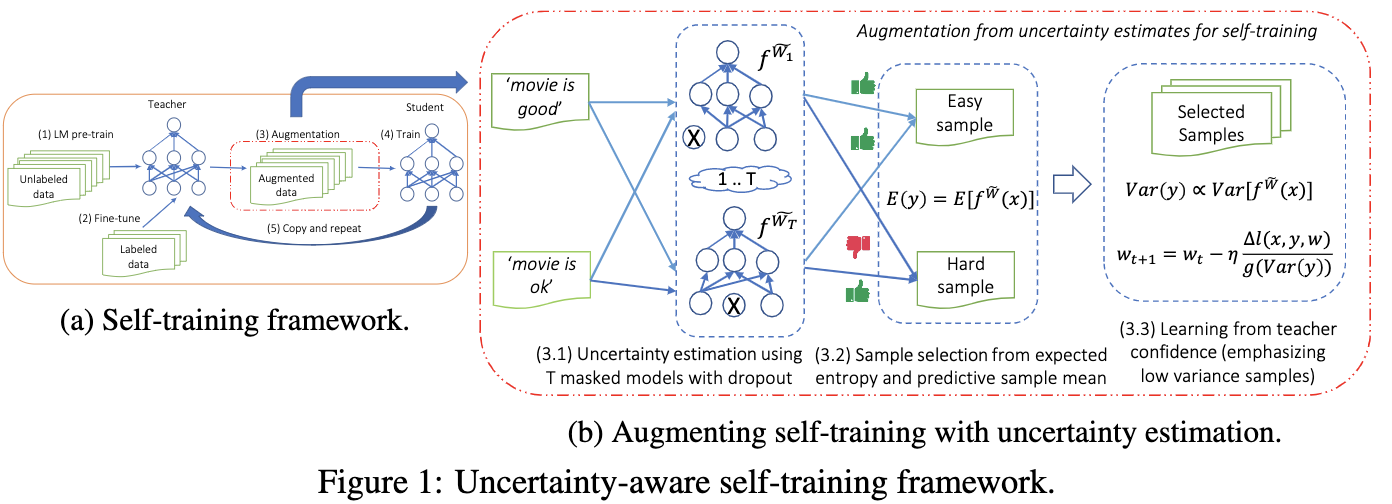5. 伪代码：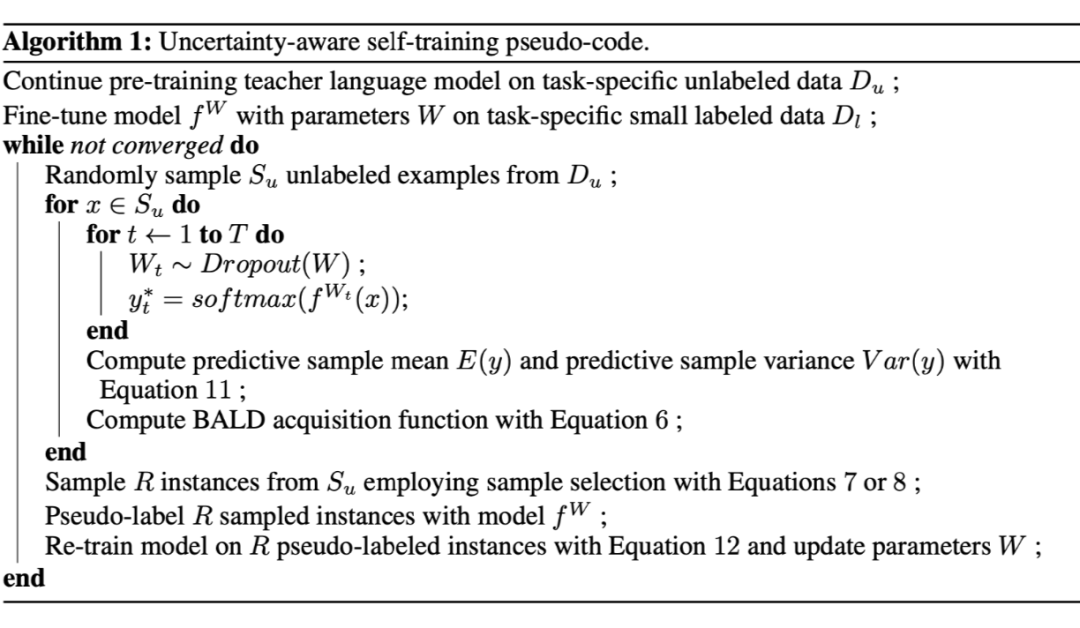#### Experiments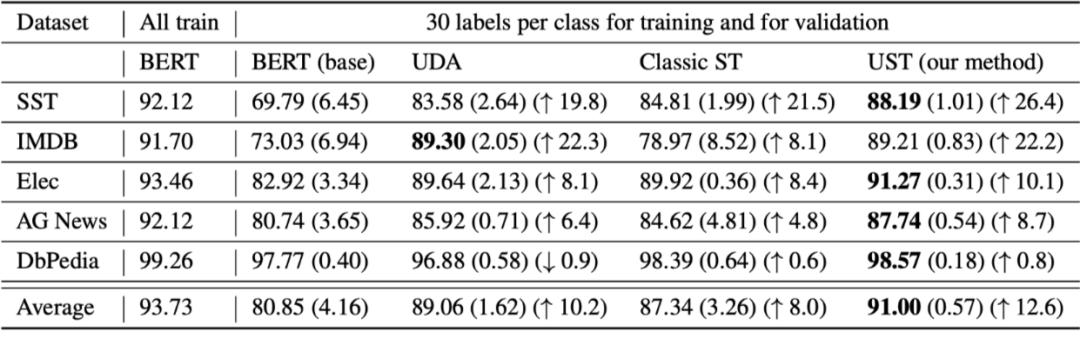### Self-Training With Noisy Student Improves ImageNet Classification

2. 这篇文章最大的亮点就在于“noisy student”，产生伪标签的过程与之前无二，但是在重新训练模型（student）的时候需要加噪声（dropout, stochastic depth and augmentation）。整体框架如下：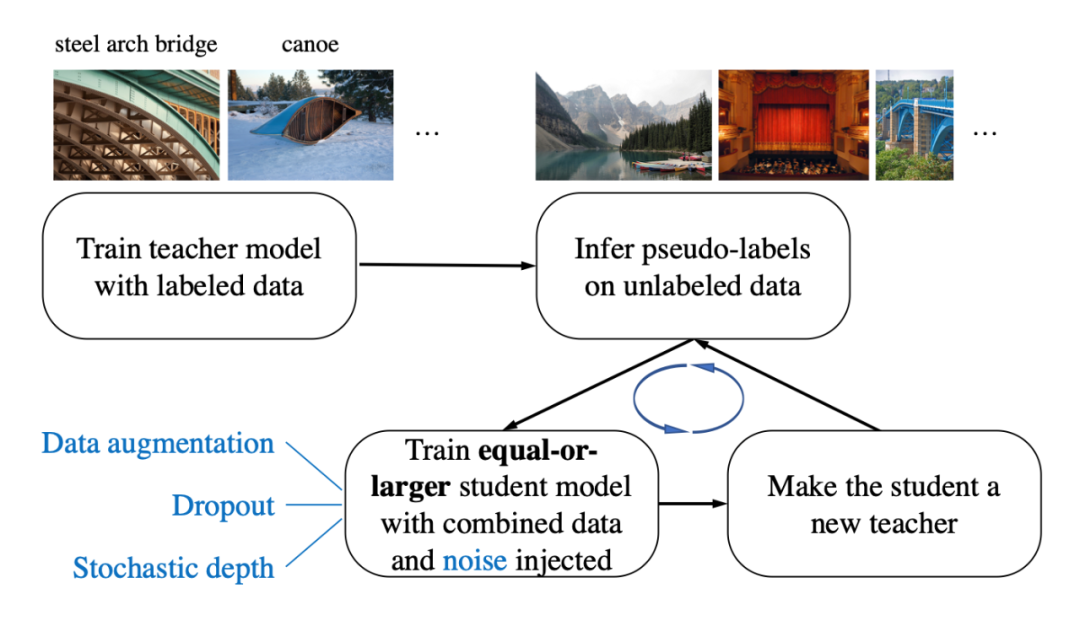3. 除此之外有两点需要注意：
1. teacher 和 student 模型架构可以相同也可以不同，但是要想使噪音学生模型学习的好，模型容量必须足够大，以拟合大量的无标注数据集。这里使用的是基准模型，即第一次的 teacher model 是 EfficentNet-B7，而 student model 是一个更大的模型，并逐渐增大直到 EfficientNet-L2。
2. 平衡数据：这是 self-training 很多都会做的一个工作，让每个类的未标记图像数量相同。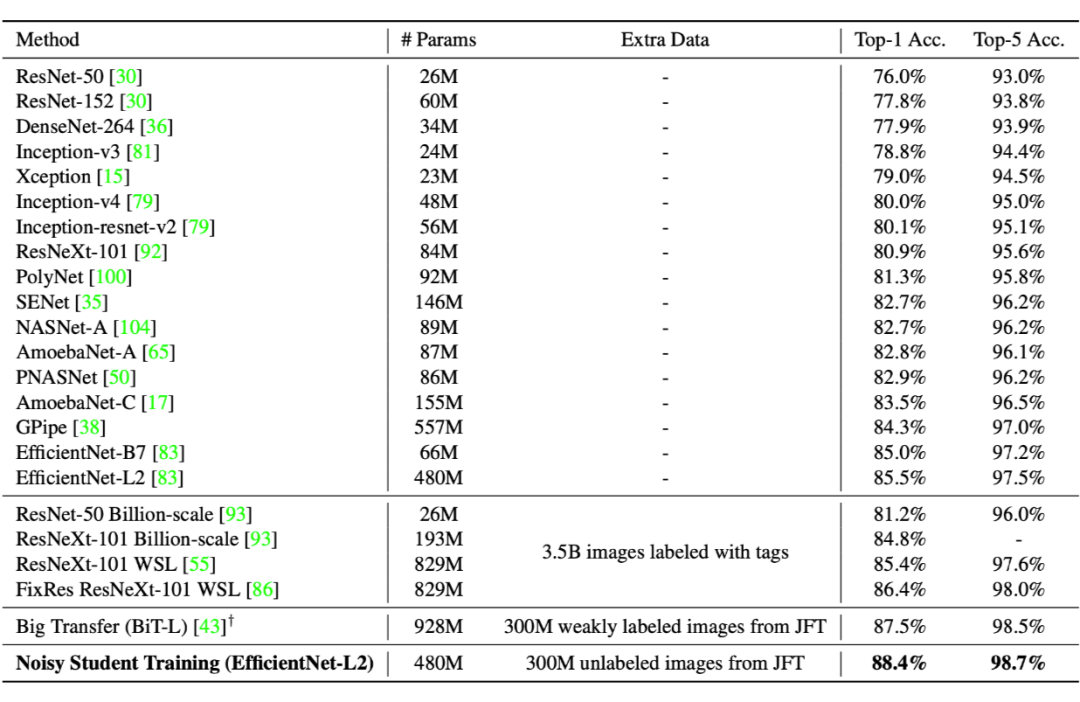### CReST: A Class-Rebalancing Self-Training Framework for Imbalanced Semi-Supervised Learning

2. 在类别不平衡的数据上施展半监督学习的研究很少，而这个问题又非常具有研究价值。该文章通过根据每个 class 样本数目对带伪标签的数据进行抽样，从而提升模型在少量样本上的表现。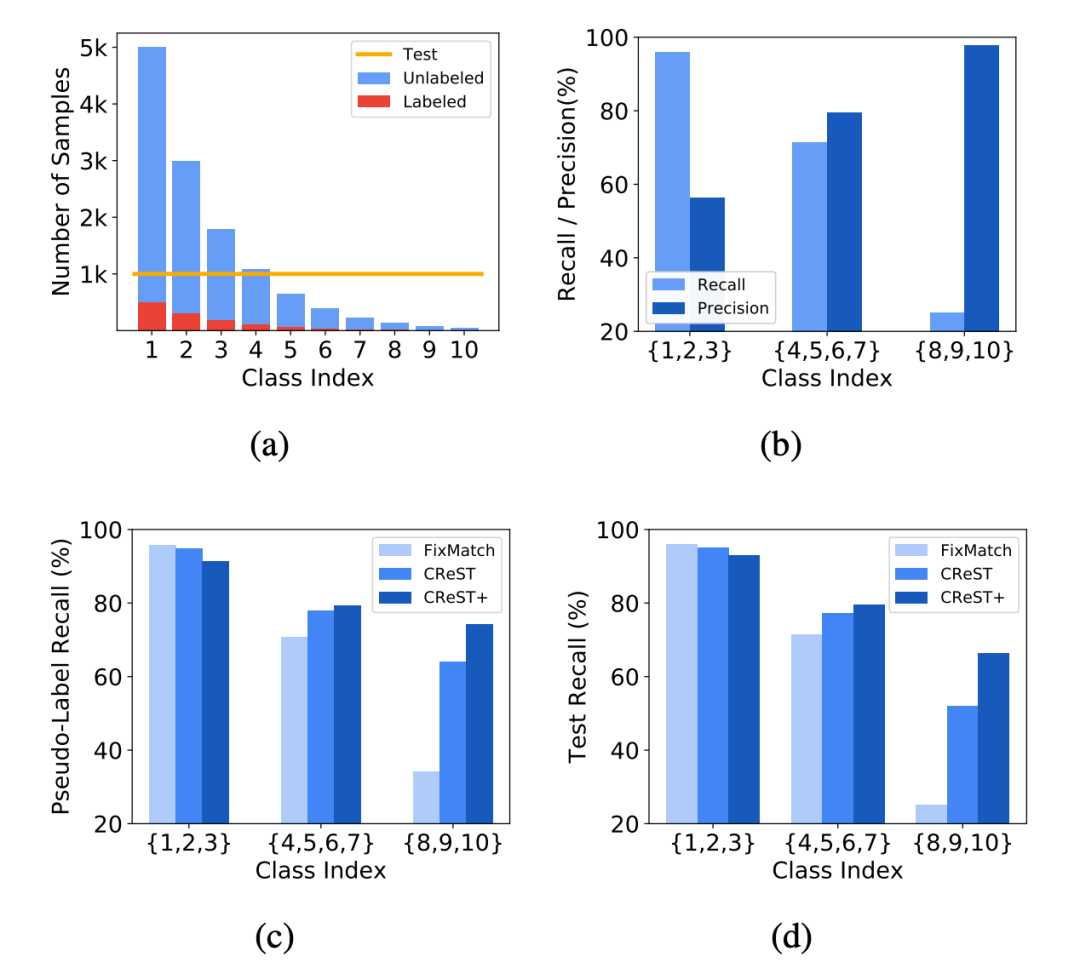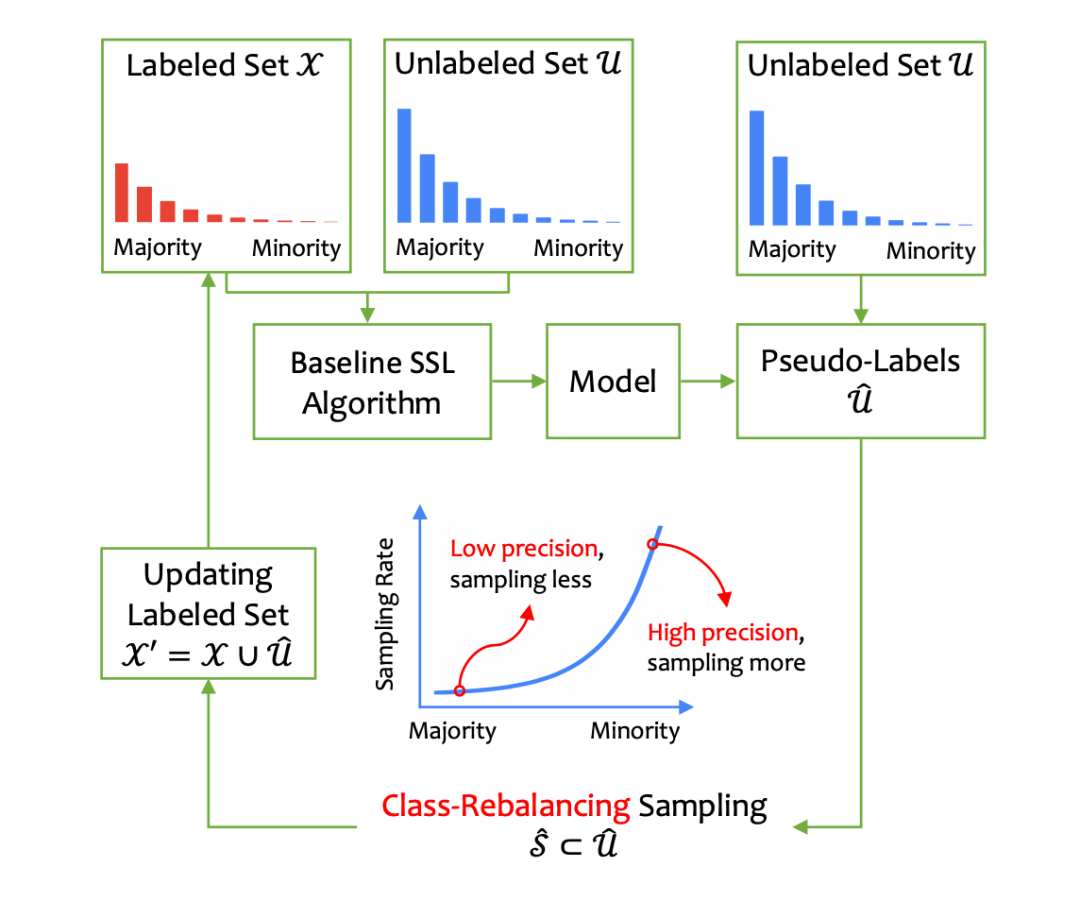$$\mu_{l}=\left(\frac{N_{L+1-l}}{N_{1}}\right)^{\alpha}$$
$\alpha>0$控制着采样频率。比如对10分类问题，被分为第 10 类的所有样本（minority class）都会被选中 $\mu_{10}=\left(\frac{N_{10+1-10}}{N_{1}}\right)^{\alpha}$（文章假设了各个类是按照样本数目从多到少排序的），而第一类的样本（majority class）只有很少一部分被选中 $\mu_{1}=\left(\frac{N_{10+1-1}}{N_{1}}\right)^{\alpha}$。这种做法有两个好处：1）因为 minority class 的预测精度都很高，因此将他们加入训练集合风险比较小；2）minority class 样本数目本来就少，对模型更加重要。

#### Experiments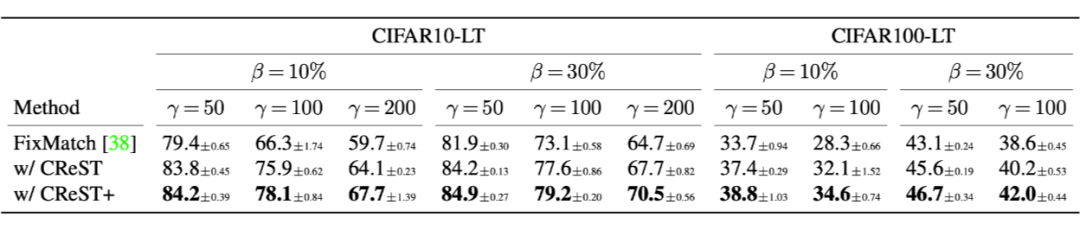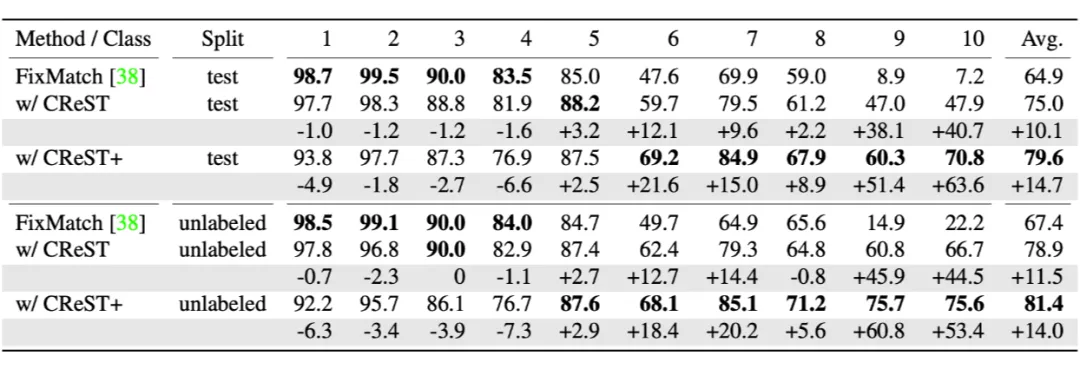### Unsupervised Data Augmentation for Consistency Training

2. 一个比较经典的self-training框架，使用监督数据计算交叉熵损失，使用无监督数据进行数据增强，然后计算增强后的样本和原始样本预测的一致性损失（KL散度，交叉熵等）。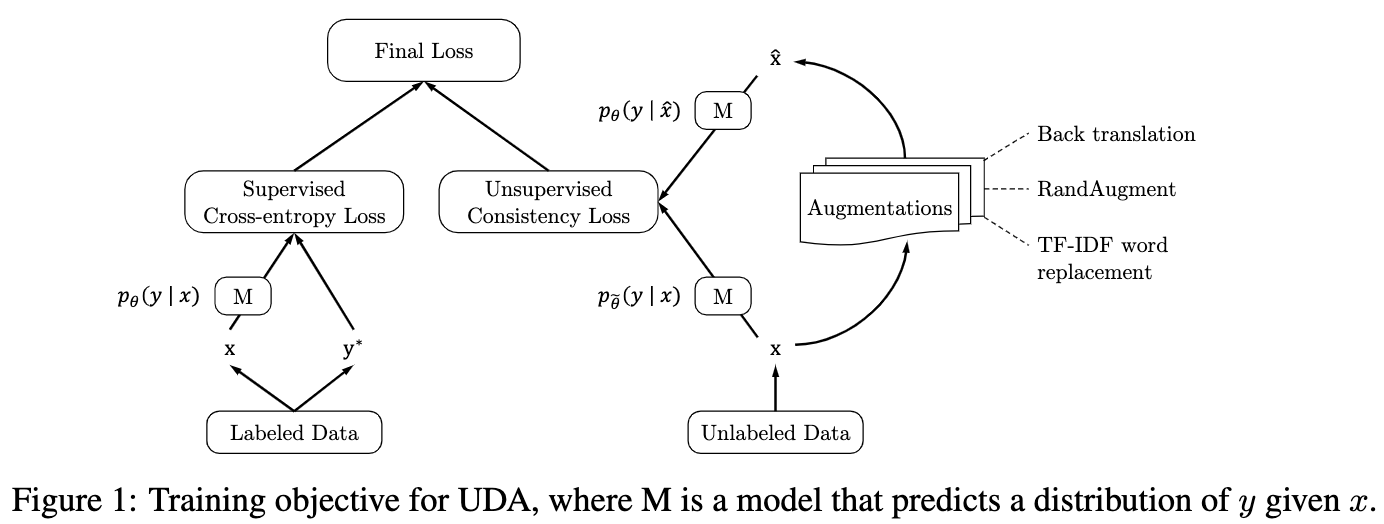### Curriculum Labeling: Revisiting Pseudo-Labeling for Semi-Supervised Learning

1. AAAI2021
2. 借鉴课程学习改善伪标注的采样过程，实验效果上与Consistency Training持平，但在OOD数据上有显著提升。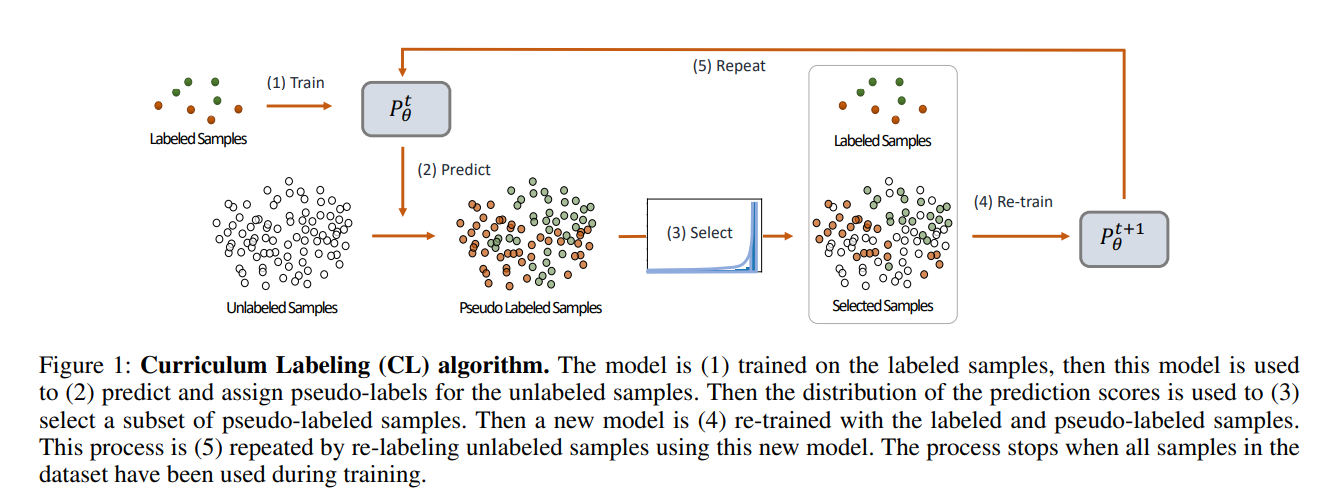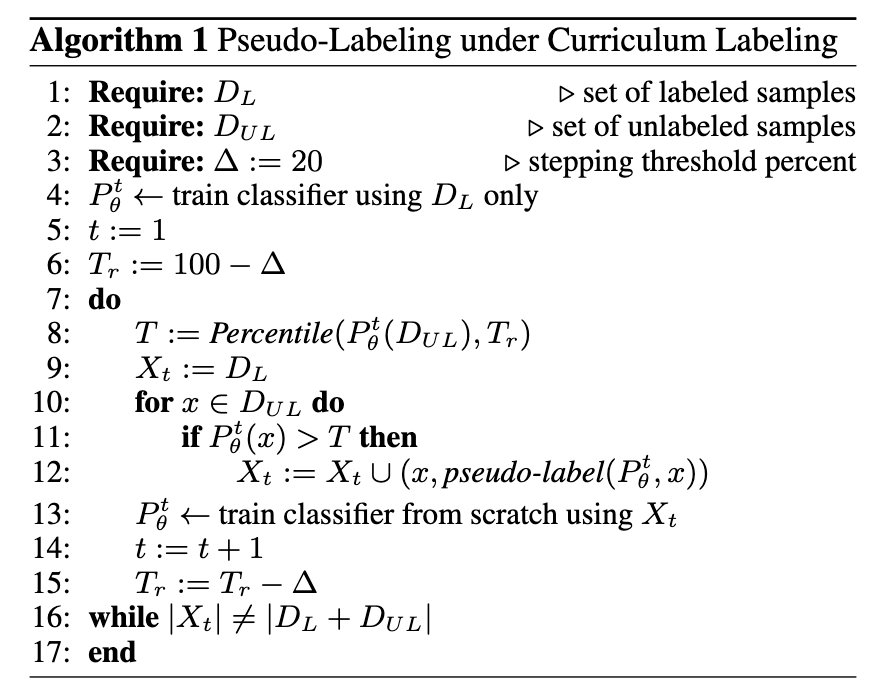### In Defense Of Pseudo-Labeling: An Uncertainty-Aware Pseudo-Label Selection Framework For Semi-Supervised Learning

1. ICLR 2021，https://zhuanlan.zhihu.com/p/350701042
2. 本文提出的Uncertainty-Aware Pseudo-Label Selection Framework (UPS)策略，正是结合了不确定性估计（Uncertainty estimation）和Negative learning的技术，不仅在传统的半监督学习任务上与一致性正则法达到旗鼓相当的水平，而且在视频半监督分类和图片多标签半监督分类领域打破了一致性正则的垄断，取得了更优的效果。